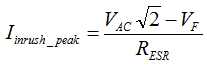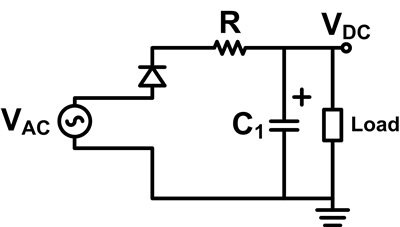# Power Tips: How to limit inrush current in an AC/DC power supply

When powering up an AC/DC power supply, a huge amount of energy transfers from the power source to the bulk capacitor. In this installment of Power Tips we examine how to limit inrush current in an AC/DC power supply.

Figure 1: Inrush current of a power supply at 120VAC/60Hz input

As a result of powering up an AC/DC power supply, you can observe an inrush current at the input of the power supply during power up transient (Figure 1). If the inrush current is too large (where the power supply sunk too much energy in a short period of time), components in the power supply, such as fuse and rectifier diodes, might be damaged. Start with the peak inrush current estimation, this blog walk you through how the inrush current could damage circuit components and give you examples of inrush current limiting circuit.

Consider the simplest AC/DC conversion, a half-wave rectifier, in Figure 2.Figure 2: A half-wave rectifier

You can estimate the peak inrush current under a no-load condition with Equation 1:VF is the forward-voltage drop of the rectifier diode and RESR is the equivalent series resistance of capacitor C1.

With VF = 1V and RESR=1Ω, the peak inrush current is 168A at a 120VAC input. This amount of inrush current is clearly over the current rating of most diodes used today. In addition, the inrush current can last for a long period of time with a large capacitance on C1, which could lead to blowing up a fuse during power-up transient (the inrush energy over the fuse I2t rating).

To avoid possible component damage caused by inrush current, an inrush-limiting circuit is generally required for an AC/DC power supply. The three types of inrush-limiting circuits designers use most are a negative temperature coefficient (NTC) thermistor, a relay, and a MOSFET bypass circuit.

NTC thermistor

An effective way to cut down the inrush current is to increase the resistance on the capacitor-charging path – inserting a resistor like that shown in Figure 3. If you insert a 2.5Ω resistor, the inrush current can easily be cut down below 70A at a 120VAC input. But if you insert a 2.5Ω resistor in a 200W power supply for current-limiting purposes, you will have over 7W power dissipation on the 2.5Ω resistor.

An efficient way to cut down the inrush is to replace the resistor with an NTC thermistor (RT1 in Figure 4). Before the power supply powers up, the NTC thermistor is cool and holding a high resistance. Hence, the high resistance can be used to limit the inrush current during power up transient.

During normal operation, the NTC thermistor is heated up and holding a low resistance – much lower than the fixed value resistor. For example, the 2.5Ω NTC thermistor used in Figure 4 becomes 0.5Ω at 100°C. The resistance reduction of NTC thermistor gives us lower power dissipation on the inrush current-limiting circuit.Figure 3: A half-wave rectifier with resistor as inrush current limiterFigure 4: Input rectifier stage of PMP5141

Relay

An NTC thermistor provides a low-cost current-limiting circuit option. However, it is still too lossy for a mid to high power level (300W – few kilowatts) power supply. Using a relay can avoid huge losses on the current-limiting circuit. Figure 5 shows the relay circuit for a 1kW power supply. The relay is initially turned off. During power up, the input current flows through a 10Ω/10W cement resistor. Once the power supply is energized, a regulated bias voltage, 12V2, turns on the relay to minimize the power dissipation on the current-limiting circuit during normal operation.Figure 5: Input rectifier stage of a 1kW power supply

MOSFET bypass circuit

Besides relay, a MOSFET bypass circuit such as the one shown in Figure 6 provides another way to lower the current-limiting-circuit power dissipation. In Figure 6, a PFC boost circuit is applied. During normal operation, the boost circuit steps up the rectified input voltage, VREC, to a higher voltage level at node B+ (380VDC nominal). The MOSFET bypass circuit detects the voltage level of B+. Once the voltage at B+ is closed to 380VDC, MOSFET Q2 is then turned on to avoid having high power dissipated on RT1.

Figure 6: Input stage of PMP9531

Please see TI’s power management reference design library for complete AC/DC power-supply designs and their current-limiting circuitries.

Parents
• Stefano and Kiril,

Thank you for point out the mistake I have made. Correct figure and description will be updated soon.

Comment
• Stefano and Kiril,

Thank you for point out the mistake I have made. Correct figure and description will be updated soon.

Children
No Data